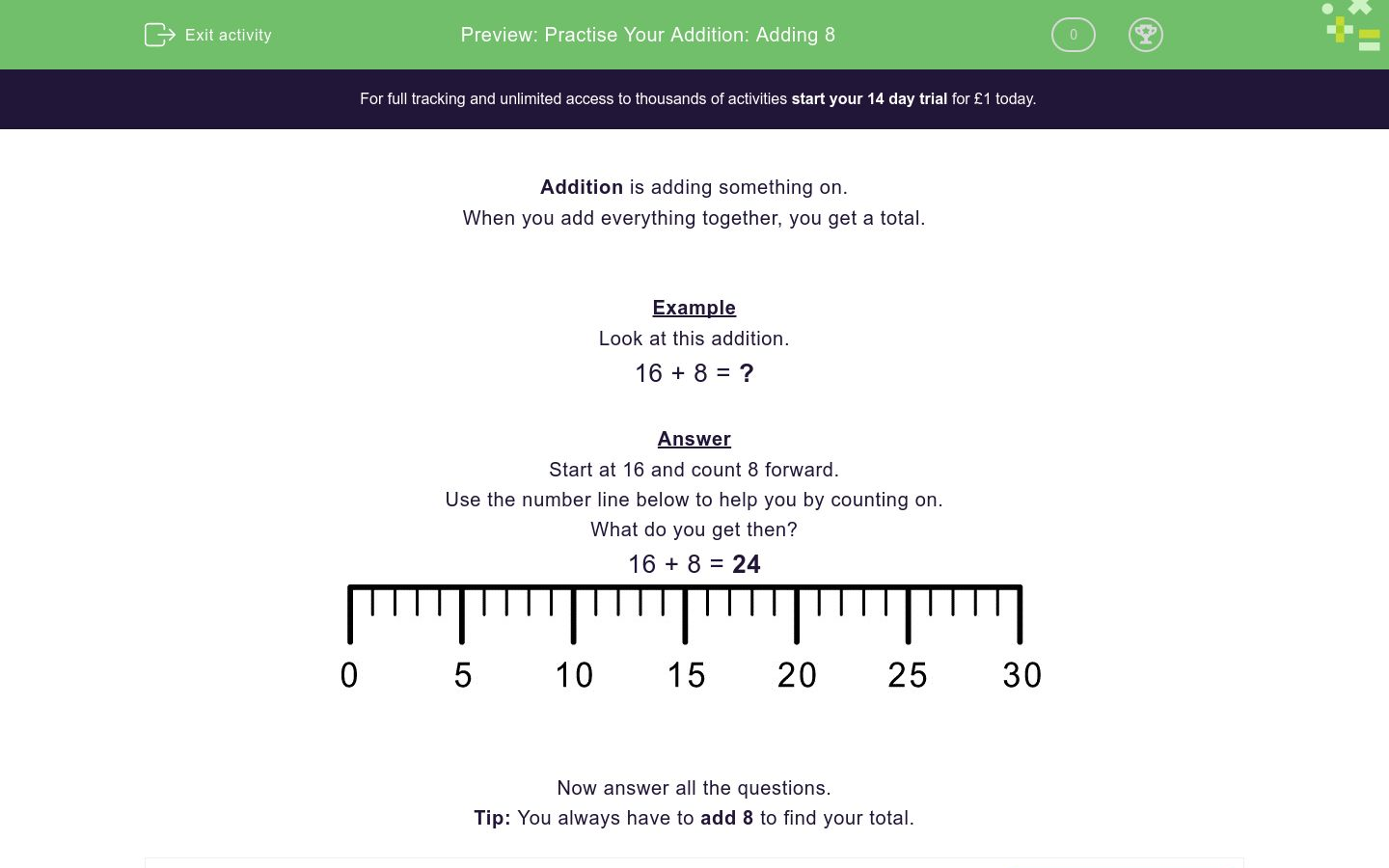### Affordable pricing from £10/monthKey stage:  KS 1

Curriculum topic:   Number: Addition and Subtraction

Curriculum subtopic:   Add and Subtract to 20

Difficulty level:### QUESTION 1 of 10

When you add everything together, you get a total.

Example

16 + 8 = ?

Start at 16 and count 8 forward.

What do you get then?

16 + 8 = 24Write the missing number in the box below:

7 + 8 = ?Write the missing number in the box below:

18 + 8 = ?Write the missing number in the box below:

3 + 8 = ?Write the missing number in the box below:

13 + 8 = ?Write the missing number in the box below:

4 + 8 = ?Write the missing number in the box below:

19 + 8 = ?Write the missing number in the box below:

17 + 8 = ?Write the missing number in the box below:

14 + 8 = ?Write the missing number in the box below:

1 + 8 = ?Write the missing number in the box below:

9 + 8 = ?• Question 1

Write the missing number in the box below:

7 + 8 = ?15
EDDIE SAYS

Method

7 + 8 = 15

Missing Number = 15

• Question 2

Write the missing number in the box below:

18 + 8 = ?26
EDDIE SAYS

Method

18 + 8 = 26

Missing Number = 26

• Question 3

Write the missing number in the box below:

3 + 8 = ?11
EDDIE SAYS

Method

3 + 8 = 11

Missing Number = 11

• Question 4

Write the missing number in the box below:

13 + 8 = ?21
EDDIE SAYS

Method

13 + 8 = 21

Missing Number = 21

• Question 5

Write the missing number in the box below:

4 + 8 = ?12
EDDIE SAYS

Method

4 + 8 = 12

Missing Number = 12

• Question 6

Write the missing number in the box below:

19 + 8 = ?27
EDDIE SAYS

Method

19 + 8 = 27

Missing Number = 27

• Question 7

Write the missing number in the box below:

17 + 8 = ?25
EDDIE SAYS

Method

17 + 8 = 25

Missing Number = 25

• Question 8

Write the missing number in the box below:

14 + 8 = ?22
EDDIE SAYS

Method

14 + 8 = 22

Missing Number = 22

• Question 9

Write the missing number in the box below:

1 + 8 = ?9
EDDIE SAYS

Method

1 + 8 = 9

Missing Number = 9

• Question 10

Write the missing number in the box below:

9 + 8 = ?17
EDDIE SAYS

Method

9 + 8 = 17

Missing Number = 17

---- OR ----

Sign up for a £1 trial so you can track and measure your child's progress on this activity.

### What is EdPlace?

We're your National Curriculum aligned online education content provider helping each child succeed in English, maths and science from year 1 to GCSE. With an EdPlace account you’ll be able to track and measure progress, helping each child achieve their best. We build confidence and attainment by personalising each child’s learning at a level that suits them.

Get started